# ASAP: fast, approximate graph pattern mining at scale

ASAP: fast, approximate graph pattern mining at scale Iyer et al., OSDI’18

I have a real soft spot for approximate computations. In general, we waste a lot of resources on overly accurate analyses when understanding the trends and / or the neighbourhood is quite good enough (do you really need to know it’s 78.763895% vs 78 ± 1%?). You can always drill in with more accuracy if the approximate results hint at something interesting or unexpected.

Approximate analytics is an area that has gathered attention in big data analytics, where the goal is to let the user trade-off accuracy for much faster results.

(See e.g. ApproxHadoop which we covered on The Morning Paper a while back).

In the realm of graph processing, graph pattern mining algorithms, which discover structural patterns in a graph, can reveal very interesting things in our data but struggle to scale to larger graphs. This is in contrast to graph analysis algorithms such as PageRank which typically compute properties of a graph using neighbourhood information.

Today, a deluge of graph processing frameworks exist, both in academia and open-source… a vast majority of the existing graph processing frameworks however have focused on graph analysis algorithms.

Arabesque explicitly targets the graph mining problem using distributed processing, but even then large graphs (1 billion edges) can take hours (e.g. 10 hours) to mine.

In this paper, we present A Swift Approximate Pattern-miner (ASAP), a system that enables both fast and scalable pattern mining. ASAP is motivated by one key observation: in many pattern mining tasks, it is often not necessary to output the exact answer. For instance, in frequent sub-graph mining (FSM) the task is to find the frequency of subgraphs with an end goal of ordering them by occurrences… our conversations with a social network firm revealed that their application for social graph similarity uses a count of similar graphlets. Another company’s fraud detection system similarly counts the frequency of pattern occurrences. In both cases, an approximate count is good enough.

ASAP outperforms Arabesque by up to 77x on the LiveJournal graph while incurring less than 5% error. It can scale to graphs with billions of edges (e.g. Twitter at 1.5B edges) and produce results in minutes rather than hours.

One major challenge is that the approximation methods used in big data analytics don’t carry over to graph pattern mining. There, the core idea is to sample the input, run the analysis over the sample, and extrapolate based on an assumption that the sample size relates to the error in the output. If we take this same idea and apply it to a foundational graph mining problem, counting triangles, it quickly becomes apparent that there is no clear relationship we can rely on between the size of the sample and the error or the speedup.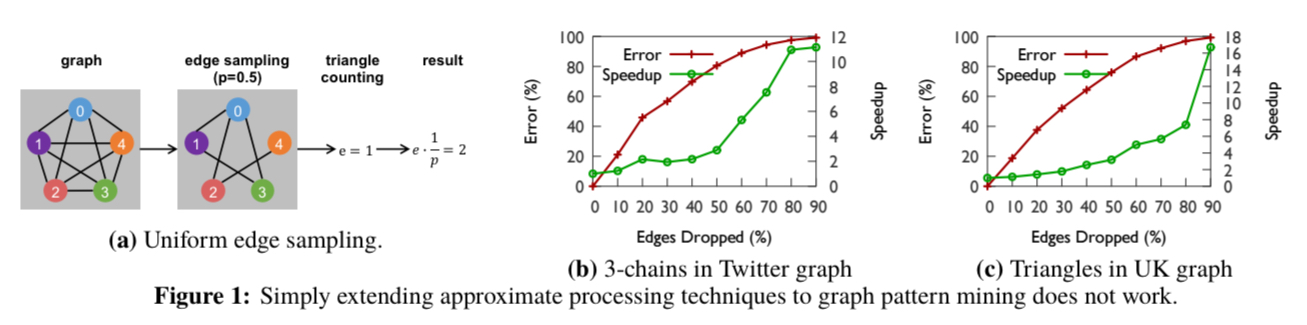We conclude that the existing approximation approach of running the exact algorithm on one or more samples of the input is incompatible with graph pattern mining. Thus, in this paper, we propose a new approach.

### Graph pattern mining and sampling

Pattern mining is the problem of finding instances of a given pattern e.g. triangles, 3-chains, cliques, motifs, in a graph. (A motif query looks for patterns involving a certain number of vertices, e.g. a 3-motif query looks for triangles and 3-chains).

A common approach is to iterate over all possible embeddings in the graph starting with a vertex or edge, filtering out those that cannot possibly match. The remaining candidate embeddings are then expanded by adding one more vertex/edge and the process repeats.

The obvious challenge in graph pattern mining, as opposed to graph analysis, is the exponentially large candidate set that needs to be checked.

Neighbourhood sampling has been proposed in the context of a specific graph pattern, triangle counting. The central idea is to sample one edge from an edge stream, and the gradually add more edges until either they form a triangle or it becomes impossible to form the pattern. Given a graph with m edges, it proceeds as follows. To perform one trial:

• Uniformly sample one edge ($l_0$) from the graph, with sampling probability 1/m.
• Uniformly sample one of l0’s adjacent edges ($l_1$) from the graph. (Note that neighbourhood sampling depends on the ordering of edges in the stream, and$l_1$ appears after$l_0$ here). If$_l0$ has c neighbours appearing in the stream after it, then the sampling probability for$l_1$ is 1/c.
• Find an edge$l_2$ appearing in the stream after$l_1$ that completes the triangle, if possible. If we do find such an edge, the sampling probability is$1/mc$.

If a trial successfully samples a triangle, we can estimate$e_i = mc$ triangles in the full graph. After conduction r trials we have an approximate result given by$\frac{1}{r}\sum_{r}e_i$.

Here’s an example using a graph with five nodes: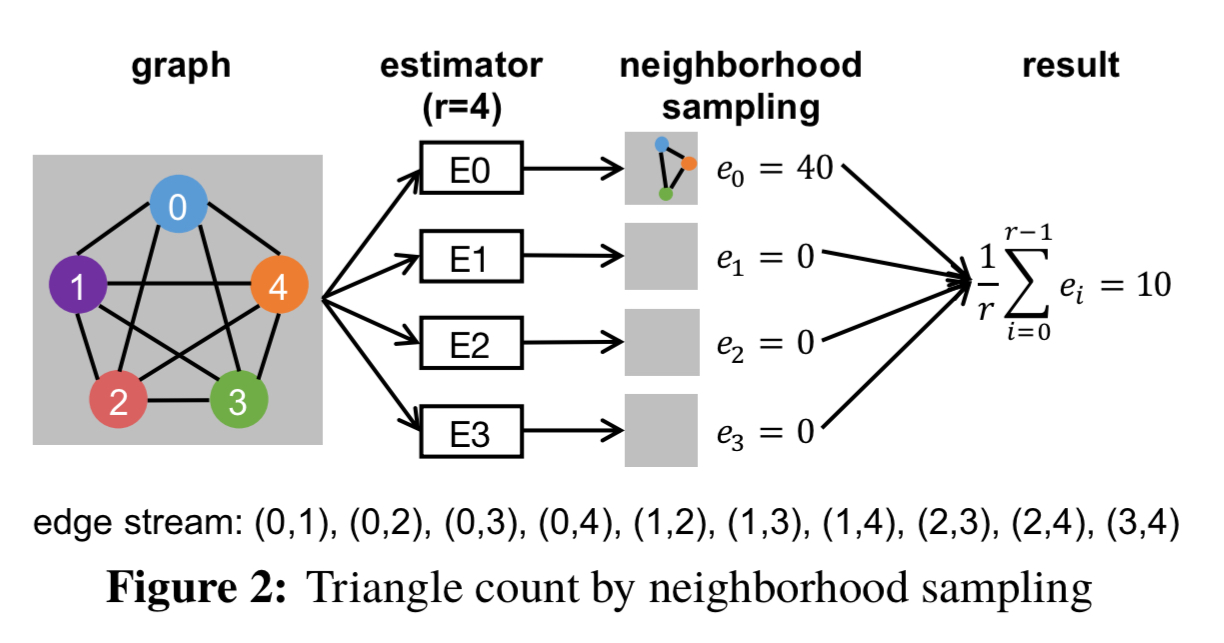### Introducing ASAP

ASAP generalises the neighbouring sampling approach outlined above to work with a broader set of patterns. It comes with a standard library of implementations for common patterns, and users can also write their own. It is designed for a distributed setting and also includes mining over property graphs (which requires predicate matching).

Users of ASAP can explicitly trade off compute time and accuracy, specifying either a time budget (best result you can do in this time) or an error budget (give me a result as quickly as you can, with at least this accuracy). Before running the algorithm, ASAP returns an estimate of the time or error bounds it can achieve. If the user approves the algorithm is run and the presented result includes a count, confidence level, and actual run time. Users may then optionally ask to output the actual embeddings of the found pattern.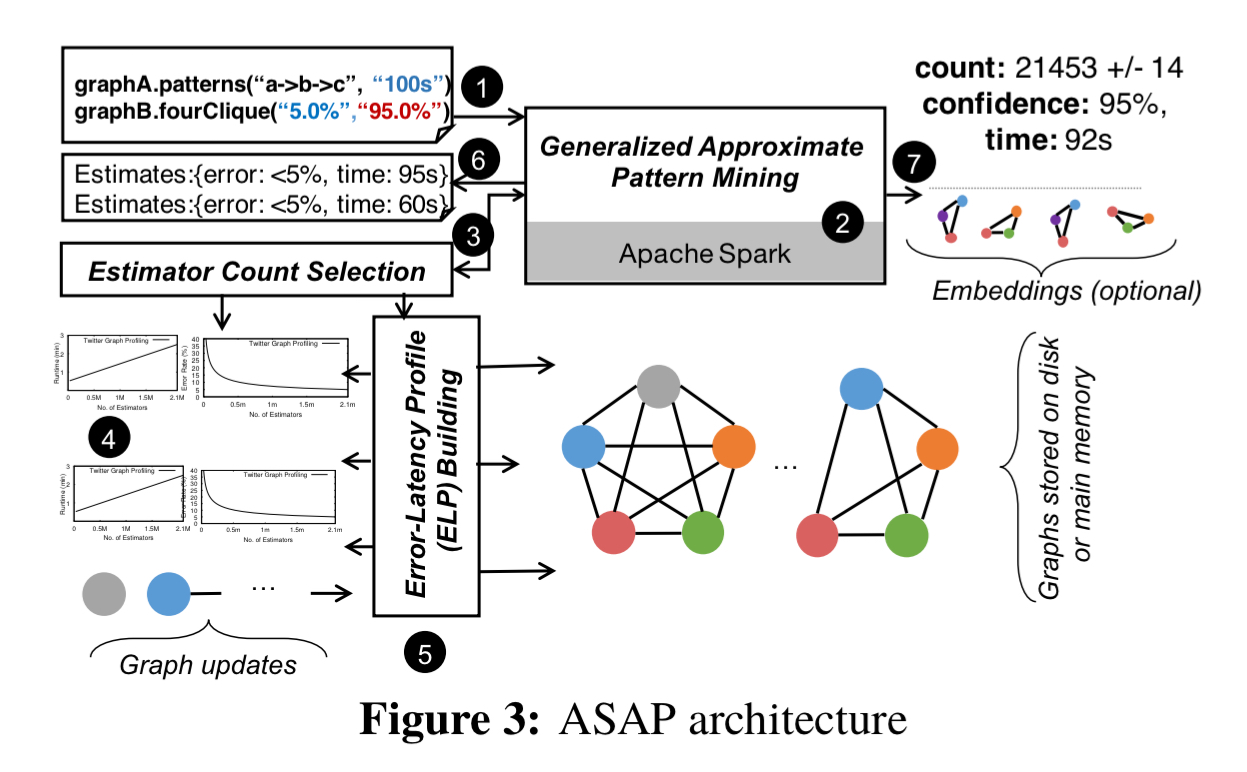To find out how many estimators it needs to run for the given bounds ASAP builds an error-latency profile (ELP). Building an ELP is fast and can be done online. For the error profile, the process begins with uniform sampling to reduce the graph to a size where nearly 100% accurate estimates can be produced. A loose upper bound for the number of estimators required is then produced using Chernoff bounds and scaled to the larger graph (see section 5.2 for details and for how the time profile is computed). For evolving graphs, the ELP algorithm can be re-run after a certain number of changes to the graph (e.g., when 10% of edges have changed).

### Approximate pattern mining in ASAP

ASAP generalises neighbourhood sampling to a two phase process: a sampling phase followed by a closing phase.

In the sampling phase, we select an edge in one of two ways by treating the graph as an ordered stream of edges: (a) sample an edge randomly; (b) sample an edge that is adjacent to any previously sampled edges, from the remainder of the stream. In the closing phase, we wait for one or more specific edges to complete the pattern.

(So in the triangle counting example above, sampling$l_0$ and$l_1$ form the sampling phase, and waiting for$l_2$ is the closing phase).

The probability of sampling a pattern can be computed from these two phases….

For a k-node pattern, the probability of detecting a pattern p depends on k and the different ways to sample using the neighbourhood sampling technique. There are four different cases for$k = 2..5$, and up to three different types of sampling possible within each case. The probability formulas for all of these are enumerated in section 4.1.1. E.g., when k=2 the probability is 1/mc as we saw previously.

When applied in a distributed setting ASAP parallelises the sampling process and then combines the outputs (MapReduce). Vertices in the graph are partitioned across machines, and several copies of the estimator task are scheduled on each machine. The graphs edges and vertices need to seen in the same order on each machine, but any order will do (‘thus, ASAP uses a random ordering which is fast and requires no pre-processing of the graph’). The output from each machine is a partial count, making the reduce step a trivial sum operation.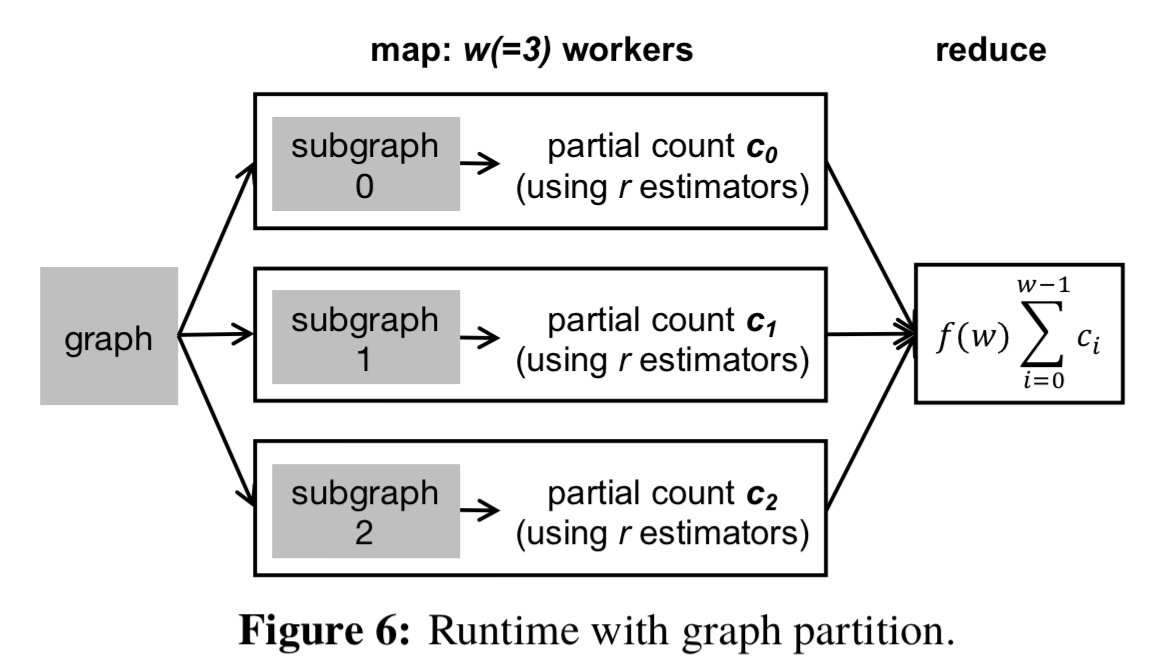For predicate matching on property graphs ASAP supports both ‘at least one’ and ‘all’ predicates on the pattern’s vertices and edges. For ‘all’ predicates, ASAP introduces a filtering phase before the execution of the pattern mining task. For ‘at least one’ predicates a first pass produces a matching list of edges matching the predicate. Then in the second pass when running the sampling phase of the algorithm every estimator picks its first edge randomly from within the matching list.

There’s a performance optimisation for motif queries (finding all patterns with a certain number of vertices) whereby common building blocks from k-node patterns can be reused.

If a user first explores the graph with a low accuracy answer (e.g. using 1 million estimators), and then wants to drill in to higher accuracy (requiring e.g. 3 million estimators) then ASAP only needs to launch another 2 million estimators and can reuse the first 1 million.

### Key evaluation results

ASAP is evaluated using a number of graphs: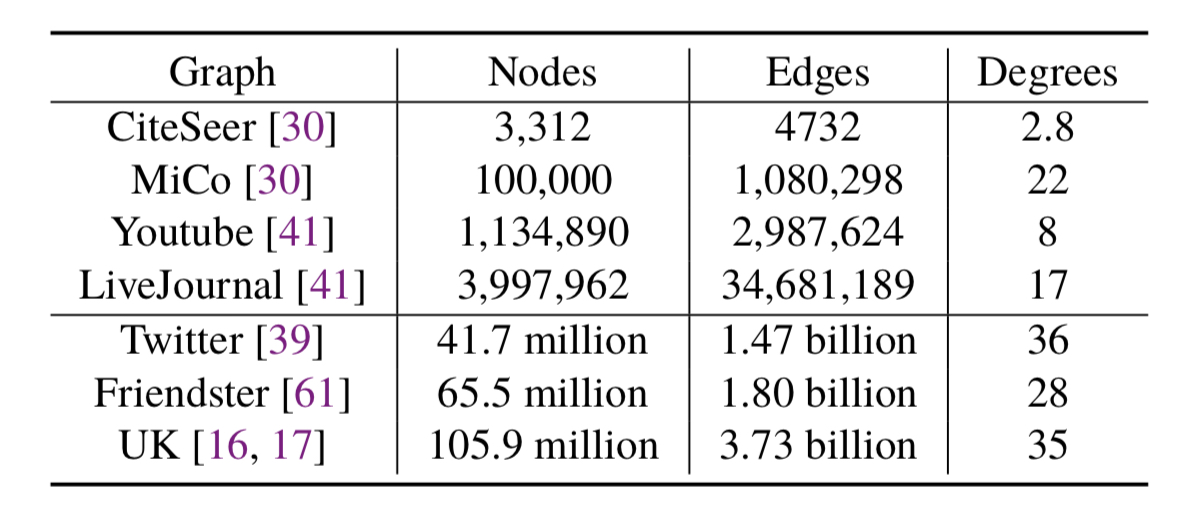Compared to Arabesque, ASAP shows up to 77x performance improvement with a 5% loss of accuracy when counting 3-motifs and 4-motifs on modest sized graphs: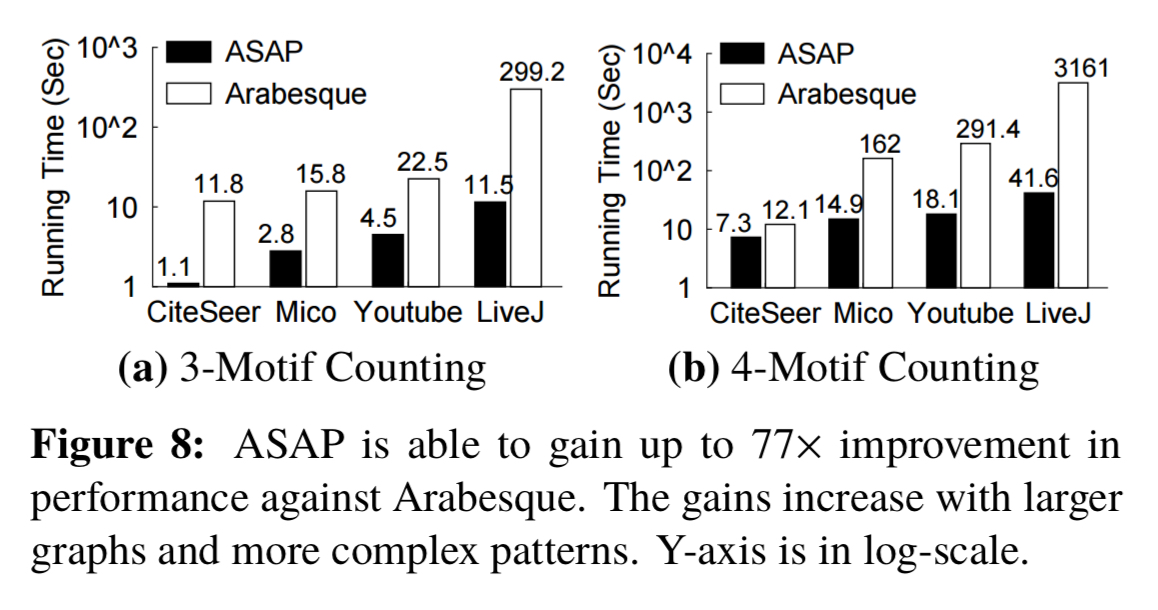On larger graphs ASAPs advantage extends up to 258x.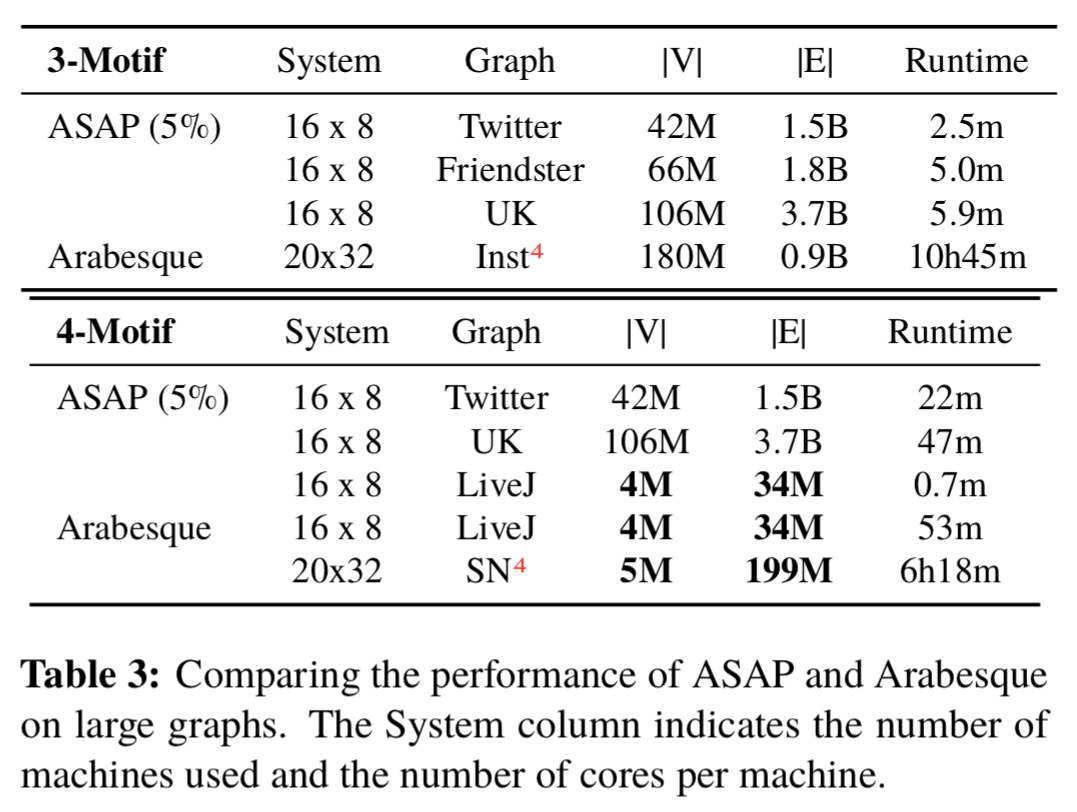The final part of the evaluation evaluates mining for 5-motifs (21 individual patterns), based on conversations with industry partners who use similar patterns in their production systems. Results for two of those patterns are shown in the table below, and demonstrate that ASAP can handle them easily (using a cluster of 16 machines each with 16 cores).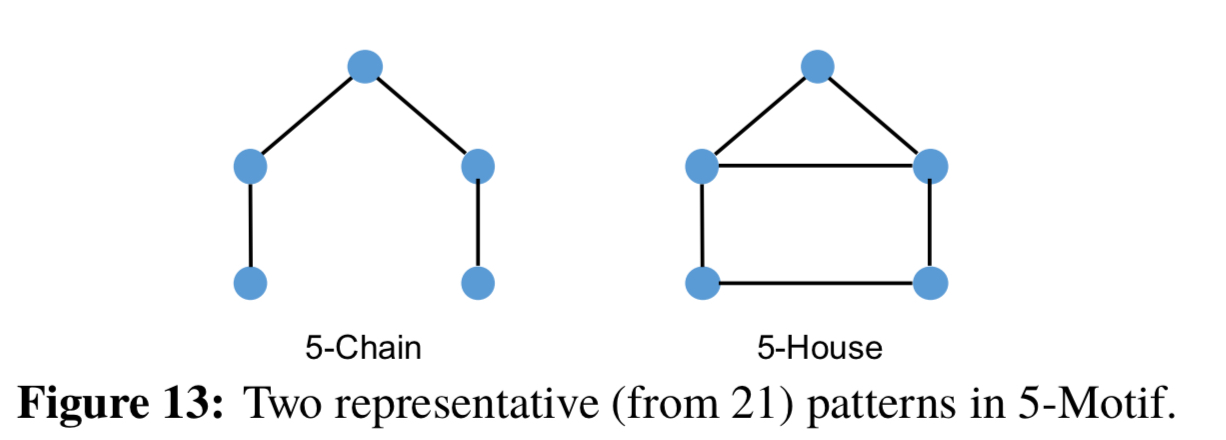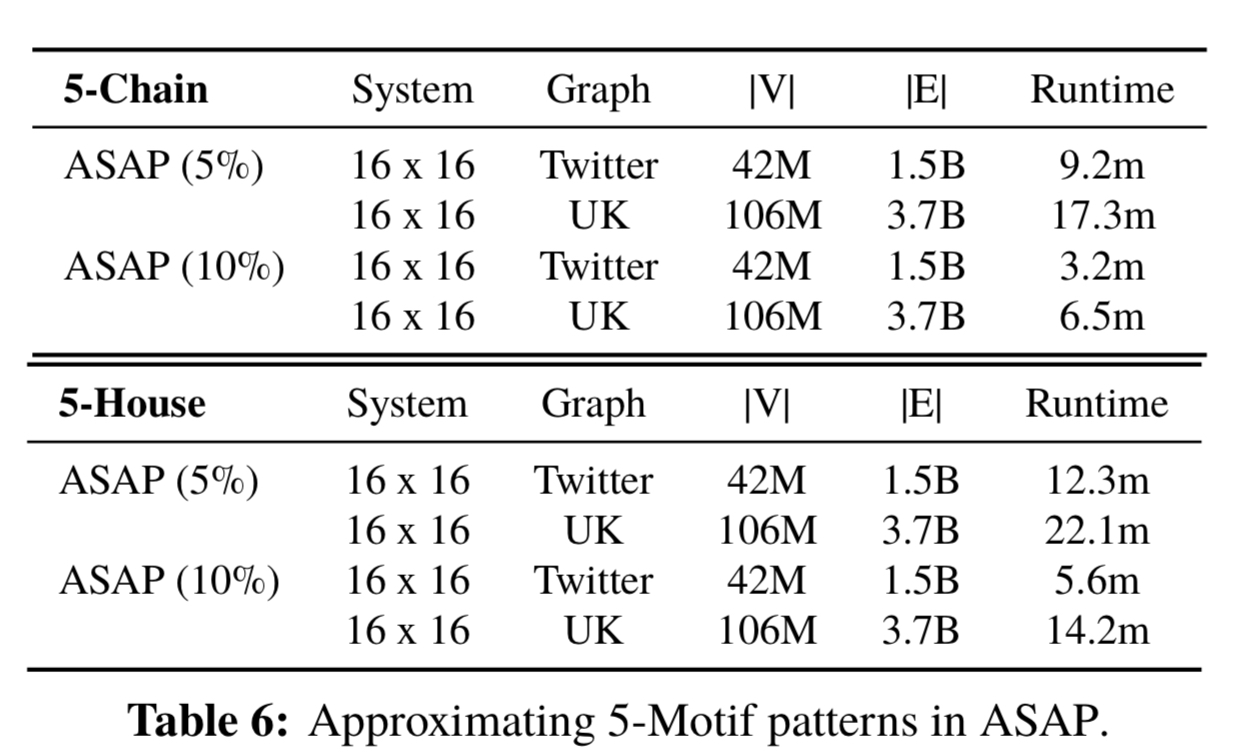Our evaluation shows that not only does ASAP outperform state-of-the-art exact solutions by more than an order of magnitude, but it also scales to large graphs while being low on resource demands.